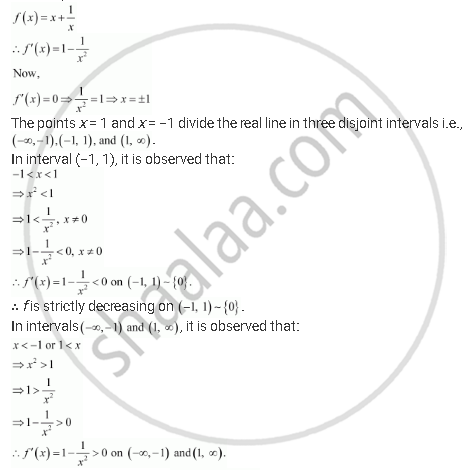Share

# Let I Be Any Interval Disjoint from (−1, 1). Prove that the Function F Given by F(X) = X + 1/X Is Strictly Increasing On I. - CBSE (Commerce) Class 12 - Mathematics

ConceptIncreasing and Decreasing Functions

#### Question

Let I be any interval disjoint from (−1, 1). Prove that the function f given by f(x) = x + 1/x is strictly increasing on I.

#### Solution

We have,∴ f is strictly increasing on (-oo, 1) and (1, oo)

Hence, function f is strictly increasing in interval I disjoint from (−1, 1).

Hence, the given result is proved.

Is there an error in this question or solution?

#### APPEARS IN

NCERT Solution for Mathematics Textbook for Class 12 (2018 to Current)
Chapter 6: Application of Derivatives
Q: 15 | Page no. 206

#### Video TutorialsVIEW ALL 

Solution Let I Be Any Interval Disjoint from (−1, 1). Prove that the Function F Given by F(X) = X + 1/X Is Strictly Increasing On I. Concept: Increasing and Decreasing Functions.
S